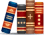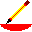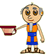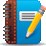# Visual Fractions Site Map

## The Complete Visual Fractions Website

### Identify Fractions

#### Description

Fractional parts of number line model are used to show the numerator and denominator.
Fractional parts of circle models are used to show the numerator and denominator.
Square and round cookies are used to demonstrate a fractional part of a group of objects
Number line models are used to show mixed numbers.
Circle models are used to show mixed numbers.
Common Core State Standards for MATHEMATICS Grade Three Use fractions along with visual fraction models to represent parts of a whole.
Common Core State Standards for MATHEMATICS Grade Three Solve problems that involve comparing fractions by using visual fraction models.

### Rename Fractions

#### Description

Number line models are used to demonstrate mixed numerals and fraction form numerals.
Circle models are used to demonstrate mixed numerals and fraction form numerals.
Number line models are used to picture renaming fractions to higher terms.
Circle models are used to picture renaming fractions to higher terms.
Number line models are used to picture renaming fractions to lowest terms.
Circle models are used to picture renaming fractions to lowest terms.
Circle models are used in an animation that demonstrates canceling.
Common Core State Standards for MATHEMATICS Grade Four Explain why a fraction a/b is equivalent to a fraction (n × a)/(n × b) by using visual fraction models

### Compare Fractions

#### Description

Number line models are used for instruction and and practice in comparing fractions.
Circle models are used for instruction and and practice in comparing fractions.
Common Core State Standards for MATHEMATICS Grade Four Record the results of comparisons with the symbols >, =, or <, and justify the conclusions, e.g., by using a visual fraction model.

#### Description

Number line models are used for instruction and practice in adding fractions.
Circle models are used for instruction and practice in adding fractions.
Number line models are used for instruction and practice in adding unlike fractions.
Circle models are used for instruction and practice in adding unlike fractions.
Number line models are used to show addition of fractions. Sums are to be written in lowest terms.
Circle models are used to show addition of fractions. Sums are to be written in lowest terms.
Common Core State Standards for MATHEMATICS Grade Four Decompose a fraction into a sum of fractions with the same denominator in more than one way, recording each decomposition by an equation. Justify decompositions, e.g., by using a visual fraction model.

### Subtract Fractions

#### Description

Number line models are used to demonstrate minuend, subtrahend, and difference.
Circle models are used to demonstrate minuend, subtrahend, and difference.
Number line models are used to demonstrate minuend, subtrahend, and difference. All differences must be written in lowest terms.
Circle models are used to demonstrate minuend, subtrahend, and difference. All differences must be written in lowest terms.

### Multiply Fractions

#### Description

A rectangular number line array is used to demonstrate multiplying fractions.
An array of circles is used to demonstrate multiplying fractions.>
A rectangular number line array is used to demonstrate multiplying fractions. Products are wrirtten in lowest terms.
A number line array is used to demonstrate multiplicative inverse.
Common Core State Standards for MATHEMATICS Grade Four a visual fraction model to represent 5/4 as the product 5 × (1/4)
Common Core State Standards for MATHEMATICS Grade Four use a visual fraction model to express 3 × (2/5) as 6 × (1/5)
Common Core State Standards for MATHEMATICS Grade Five use a visual fraction model to show (2/3) × 4 = 8/3, and create a story context for this equation

### Divide Fractions

#### Description

Number lines models are used to show the dividend, divisor, and quotient to give instruction and practice in dividing fractions.
Circle models are used to show the divisor, dividend, and quotient to give instruction and practice in dividing fractions.
Number lines models are used for instruction and practice in dividing fractions. All answers are to be written in lowest terms.
Circle models are used for instruction and practice in dividing fractions. All answers are to be written in lowest terms.
Common Core State Standards for MATHEMATICS Grade Six create a story context for (1/3) ÷ 4, and use a visual fraction model to show the quotient.
Common Core State Standards for MATHEMATICS Grade Six create a story context for 4 ÷ (1/5), and use a visual fraction model to show the quotient.

##VISUAL FRACTIONS INVESTIGATE

### Investigate Important Fractions Concepts

#### Introducing

Investigate Identify Investigate Identify whole number, numerator, denominator, fraction bar
Investigate Mixed to Fraction Investigate Mixed to Fraction fraction form, mixed form
Investigate Fraction to Mixed Investigate Fraction to Mixed fraction form, mixed form
Investigate Rename Higher Investigate Rename Higher higher terms, identity
Investigate Rename Lower Investigate Rename Lower lower terms, lowest terms, identity
Investigate Compare Investigate Compare common denominator, least common denominator, like fractions, unlike fractions
Investigate Subtract Investigate Subtract minuend subtrahend, difference
Investigate Multiply Investigate Multiply factor, product, reciprocal, multiplicative inverse, identity, invert
Investigate Divide Investigate Divide dividend, divisor, quotient

##VISUAL FRACTIONS DESIGNER

### Identify Fractions Designer

#### Description

Input numerator and denominator to make your own fraction examples. All examples are modeled with number lines.
Input numerator and denominator to make your own fraction examples. All examples are modeled with circles.
Input numerator, and denominator to to see how a mixed number is formed. All examples are modeled with number lines.
Input numerator, and denominator to to see how a mixed number is formed. All examples are modeled with circles.
Input numerator and denominator to make a fractions of a group of objects.

### Rename Fractions Designer

#### Description

Input whole number, numerator, and denominator to make your fraction form(improper) fracton examples. All examples are modeled with number lines.
Input whole number, numerator, and denominator to make your fraction form(improper) fracton examples. All examples are modeled with circles.
Input numerator, denominator, and factor to make your rename to higher terms examples. All examples are modeled with number lines.
Input numerator, denominator, and factor to make your rename to higher terms examples. All examples are modeled with circles.
Input numerator, denominator, and multiplyier to make your rename to lower terms examples. All examples are modeled with number lines.
Input numerator, denominator, and multiplyier to make your rename to lower terms examples. All examples are modeled with circles.

### Compare Fractions Designer

#### Description

Input numerator, and denominator to make your own compare fractions examples. All examples are modeled with number lines.
Input numerator, and denominator to make your own compare fractions examples. All examples are modeled with circles.

#### Description

Input addends to make your own addition of fractions examples. All examples are modeled with number lines.

### Subtract Fractions Designer

#### Description

Input minuend and subtrahend to make your subtraction examples. All examples are modeled with number lines.
Input minuend and subtrahend to make your subtraction examples. All examples are modeled with circles.

### Multiply Fractions Designer

#### Description

Input factors to make your multiply fraction examples. All examples are modeled with a rectangular array.
Input factors to make your multiply fraction examples. All examples are modeled with an array of circles.
More suggestions for multiply fractions designer. See how you can demonstrate some important algebraic ideas.

### Divide Fractions Designer

#### Description

Input dividend and divisor to make your own divide fractions examples. All examples are modeled with number lines.
Input dividend and divisor to make your own divide fractions examples. All examples are modeled with circles.

##VISUAL FRACTIONS GAMES

### Have Fun with Visual Fractions Games

#### Description

Find Grampy Use the numerator to find Grampy as he hides a fractional distance along a hedge.
Find Grampy-Strict Grammy will point to help you find Grampy who is hiding a fractional distance along a hedge. Answers must be in lowest terms.
Find Grammy Grampy uses two words to help you find Grammy who is hiding a fractional distance along a hedge.
Cookies for Grampy Addition, subtraction, and multiplication of fractions are used in this fraction game. Students build whole circles with fraction parts.
Egyptian Barley Cakes Use unit fractions the Ancient Egyptian way to pay workers with barley cakes.
A decimal fractions game that finds decimal positions along a number line to thousandths.
Ancient Egyptian Cookies Similar to Cookies for Grampy game but unit fractions are used.
Help feed a bee by moving a blossom along a circular dial. Addition and subtraction of fractions are demonstrated.
Weigh three objects using decimal weights.
Find decimal positions along a linear dial by weighing five different characters.
Find decimal positions along a linear dial by finding the total weight of five different characters.

##VISUAL FRACTIONS WORKSHEETS

### More Practice with Visual Fractions Worksheets

#### Key Words and Suggested Instruction:

Identify Fractions (8 worksheets)

whole number, numerator, denominator, fraction bar

How to Identify Fractions

Numerator and Denominator (5 worksheets)

whole number, numerator, denominator, fraction bar

How to Identify Fractions

Rename Fraction to Mixed (7 worksheets)

fraction form, mixed form, improper

How to Rename from Fraction Form to Mixed Form

Rename Mixed to Fraction (7 worksheets)

fraction form, mixed form, improper

How to Rename from Mixed Form to Fraction Form

Lines to Circles (5 worksheets)

whole number, numerator, denominator, fraction bar

How To Identify Fractions

Circles to Lines (5 worksheets)

whole number, numerator, denominator, fraction bar

How to Identify Fractions

Rename to Higher Terms (7 worksheets)

higher terms, reduce, rename, renaming, identity, greatest common factor

How to Rename to Higher Terms

Rename to Lower Terms (8 worksheets)

lowest terms, reduce, rename, renaming, identity, Common factor, greatest common factor

How to Rename to Lower Terms

Compare (9 worksheets)

numerator, denominator, unlike denominator, common denominator, least common multiple

How to Compare Fractions

Subtract (9 worksheets)

minuend, subtrahend, difference

How to Subtract Fractions

Multiply (9 worksheets)